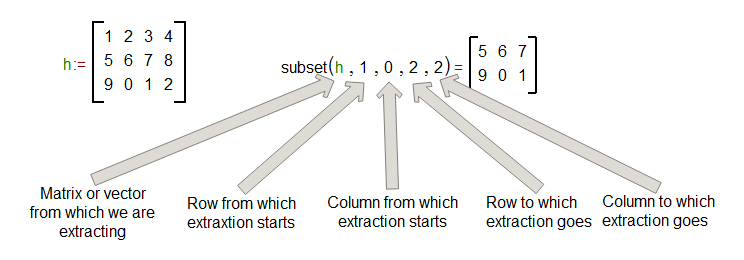# Vectors and Matrix

There are two ways to create vectors and matrices in MatDeck.

The first one: place cursor in the canvas and use the Insert Matrix option from Math tab.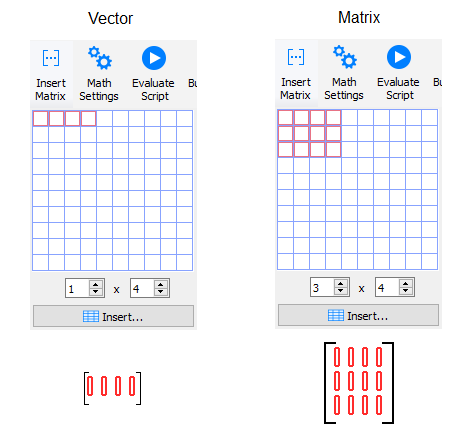The second one: use a vector or matrix function that will create a 2×1 vector or a 2×2 matrix.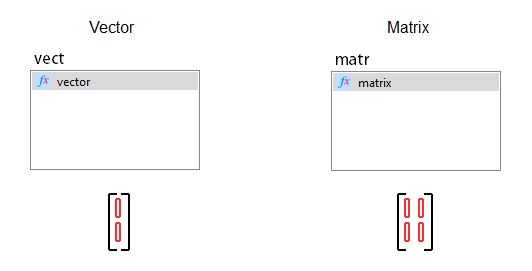To create a new column right from the current cursor position column, use the Space key on your keyboard. Remember that for this action, the node where the cursor is placed has to be empty.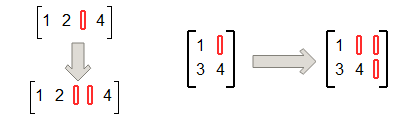To create a new row under the current cursor position row, use the Enter key on your keyboard. For this action also, node where the cursor is placed has to be empty.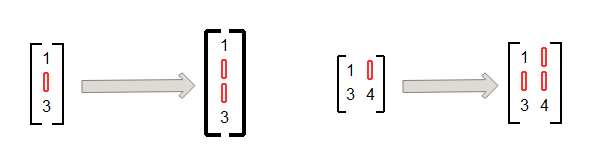To delete the current cursor position column, use Ctrl + Space keys. Node where the cursor is placed has to be empty.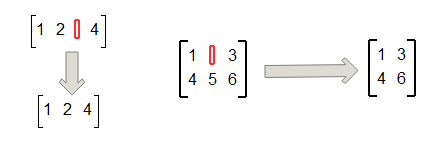To delete the current cursor position row, press Ctrl + Enter. Again, make sure that the node where the cursor is placed is empty.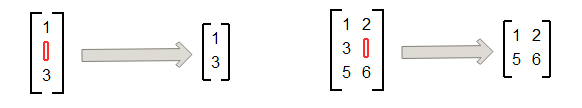Now we are going to explore functions for vector and matrix manipulations. Create three vectors and two matrix and place them in variables. To join them one beside another , use function join matrix columns.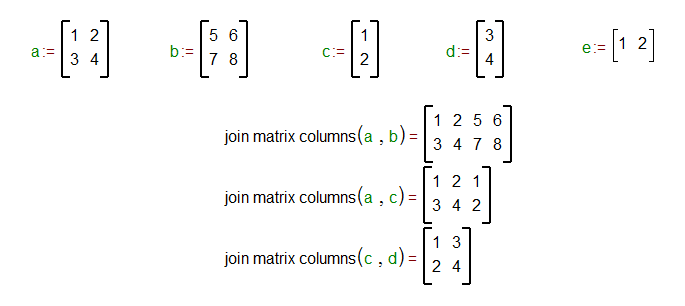To join them one above the other use function join matrix rows.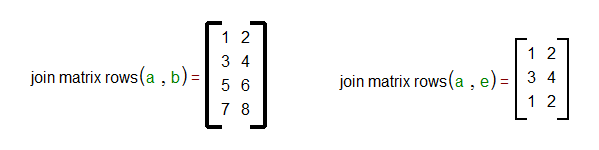To extract one column from vector or matrix use the function column2vector. Second argument is the position of column (zero indexed).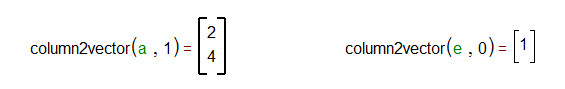To extract one row from vector or matrix, use the function row2vector. Second argument is the position of the row (zero indexed).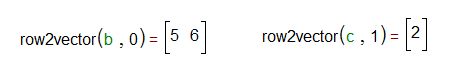To assign a specific value to a specific position of vector or a matrix, use function set value at.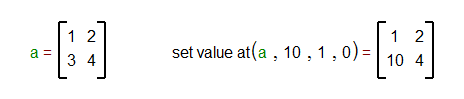Third argument of set value at function is the position of row and the fourth argument is the position of column of inserted matrix.

To create a matrix from two column vectors use function vec2mat column.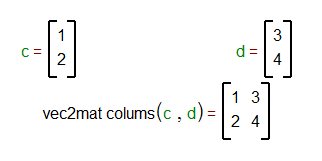To create a matrix from two row vectors use the function called vec2mat rows.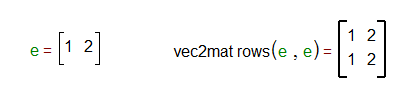Last function that we are going to use in this lesson is subset. With the subset function, you can extract a part of a vector of matrix.